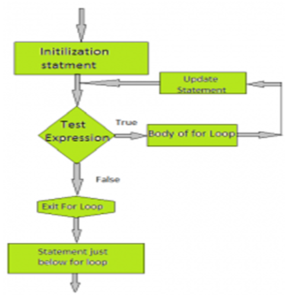# For Loop in C | For Loop Syntax | For Loop Program

In this article for loop in c we give the information about the for loop is another entry-controlled loop that provides a more concise loop control structure .

#### for loop in c:

The for loop is another entry-controlled loop that provides a more concise loop control structure .

For loop Syntax:

for(initialization; test-condition; increment/decrement)
{

Body of the loop.
}

The implementation of the statement is as follows:

Using assignment statements like i = 0 and count = 0, control variables are initialized first. The i and count variables are known as loop-control variables.

The value of the control variable is checked using test-condition. Test-mode is a relational expression, such as i <10, which determines when the loop exits. If the condition is true, the main part of the loop will be executed; Otherwise the loop is terminated and the execution continues with a statement following the loop immediately.

When the main part of the loop is executed, the last statement in the loop is evaluated and transferred back to the control statement. Now the control variable is assigned an assignment statement like i = i + 1.

And the new value of the control variable is re-checked to see if it meets the loop condition. When the condition is met, the main part of the loop is reactivated. This process continues until the value of the control variable fails to complete the test-condition.

For Loop Flowchart:For loop example in c:

// Program to calculate the sum of first n natural numbers

#include <stdio.h>

#include<conio.h>
void main()
{
int num, count, sum = 0;

clrscr();

printf(“Enter a positive integer: “);

scanf(“%d”, &num);

for(count = 1; count <= num; ++count)
{
sum += count;
}

printf(“Sum = %d”, sum);      getch();
}

Out/Put:

Enter a positive integer: 10

Sum = 55

#### Some More:

POP- Introduction to Programming Using ‘C’

DS – Data structure Using C

OOP – Object Oriented Programming

Java Programming

DBMS – Database Management System

RDBMS – Relational Database Management System